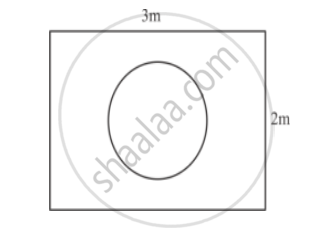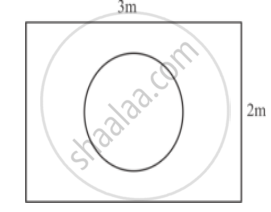Advertisement Remove all ads

# Suppose You Drop a Tie at Random on the Rectangular Region Shown in the Given Figure. What is the Probability that It Will Land Inside the Circle with Diameter 1 M? - Mathematics

Sum

Suppose you drop a tie at random on the rectangular region shown in the given figure. What is the probability that it will land inside the circle with diameter 1 m?Advertisement Remove all ads

#### Solution

Suppose you drop a tie at random on the rectangular region shown in figureProbability that it will land in inside the circle of diameter 1m Total area of circle with diameter 1 m

"Area of circle with diameter 1m"=pi(1/2)^2

=pi/4 m^2

"Area of reactangle" =3xx2

 =6m^2

"We know that PROBABILITY" = "Number of favourable event"/"Total number of event"

Hence probability that the tie will land in the circle is pi/(4/6)=pi/24

Is there an error in this question or solution?
Advertisement Remove all ads

#### APPEARS IN

RD Sharma Class 10 Maths
Chapter 16 Probability
Exercise 16.2 | Q 1 | Page 33
Advertisement Remove all ads
Advertisement Remove all ads
Share
Notifications

View all notifications

Forgot password?